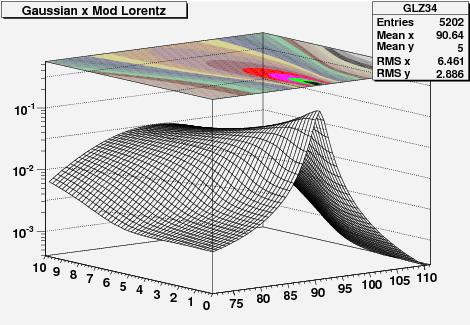## Result now, explanation later December 18, 2008

Posted by dorigo in personal, physics, science.
Tags: , , ,

Tonight I feel accomplished, since I have completed a crucial update of the cornerstone of the algorithm which provides the calibration of the CMS momentum scale. I have no time to discuss the details tonight, but I will share with you the final result of a complicated multi-part calculation (at least, for my mediocre standards): the probability distribution function of measuring the Z boson mass at a certain value$M$, using the quadrimomenta of two muon tracks which correspond to an estimated mass resolution$\sigma_M$, when the rapidity of the Z boson is$Y_Z$.

The above might -and should, if you are not a HEP physicist- sound rather meaningless, but the family of two-dimensional functions$P(M,\sigma_M)_Y$ is needed for a precise calibration of the CMS tracker. They can be derived by convoluting the production cross-section of Z bosons$\sigma_M$ at a given rapidity$Y$ with the proton’s parton distribution functions using a factorization integral, and then convoluting the resulting functions with a smearing Gaussian distribution of width$\sigma_M$.

Still confused ? No worry. Today I will only show one sample result – the probability distribution as a function of$M$ and$\sigma_M$ for Z bosons produced at a rapidity$2.8< |Y| <2.9$, and tomorrow I will explain in simple terms how I obtained that curve and the other 39 I have extracted today.In the three-dimensional graph above, one axis has the reconstructed mass of muon pairs$M$ (from 71 to 111 GeV), the other has the expected mass resolution$\sigma_M$ (from 0 to 10 GeV). The height of the function is the probability of observing the mass value$M$, if the expected resolution is$\sigma_M$. On top of the graph one also sees in colors the curves of equal probability displayed on a projected plane. It will not escape to the keen eye that the function is asymmetric in mass around its peak: that is entirely the effect of the parton distribution functions…1. JJ - December 18, 2008

It would be interesting to see the effect of the PDF uncertainty on this plot (and how sensitive it is the the uncertainties). Indeed, around the Z peak, the CTEQ6.1 uncertainty is ~5%.dorigo - December 19, 2008

Hi James,

indeed, these are CTEQ6 PDF, and my program does use the 40 eigenvector variations for an estimate of the uncertainty. However, so far I have not ran the full grid of points for the systematics, because it is very time-expensive. I however think that while the normalization does have a sizable uncertainty, the shape is not affected too much.
Another thing to note is that this is a LO calculation of the factorization integral, and so I must use LO PDF. The eigenvectors are known for the NLO sets. They can be used for a eyeballing of the uncertainty all the same, but the right thing to do is rather to compare the results I get to those I get with MRST sets. I will post about that when I get there…

Cheers,
T.2. JJ - December 19, 2008

Ah that’s interesting. So, I take it then that your method is sensitive to shape and not to normalisation then?dorigo - December 19, 2008

Well, yes, I only care about the shapes. In fact, the probability distribution shown in the plot is normalized such that the integral over the mass range, for any given resolution, is unity.
I just checked a few things about the MRST04 set I want to use for some comparisons, in http://xxx.lanl.gov/PS_cache/hep-ph/pdf/9907/9907231v1.pdf , and indeed they speak of 5% differences in cross-section; but they also admit they had some error in earlier calculations.

Cheers,
T.3. Tom - December 19, 2008

[Aesthetic question]
Hey! How do you do, using ROOT, to draw that contour projection on the top plane of your 3D box frame????dorigo - December 20, 2008

TH2D * A = new TH2D (“A”, “Goofy”, 100, 0., 100., 100, 0., 100.);
….
A->Draw(“SURF3”);

Cheers,
T.

4. English Language Summaries « Life as a Physicist - December 19, 2008

[…] the best summaries of this nature I know about are on a blog – Tomasso’s, specifically (e.g. here and here for recent […]

Sorry comments are closed for this entry